Скачать презентацию What role should probabilistic sensitivity analysis play in

7ab91895781548bf7625e2181a907c59.ppt

• Количество слайдов: 27What role should probabilistic sensitivity analysis play in SMC decision making? Andrew Briggs, DPhil University of Oxford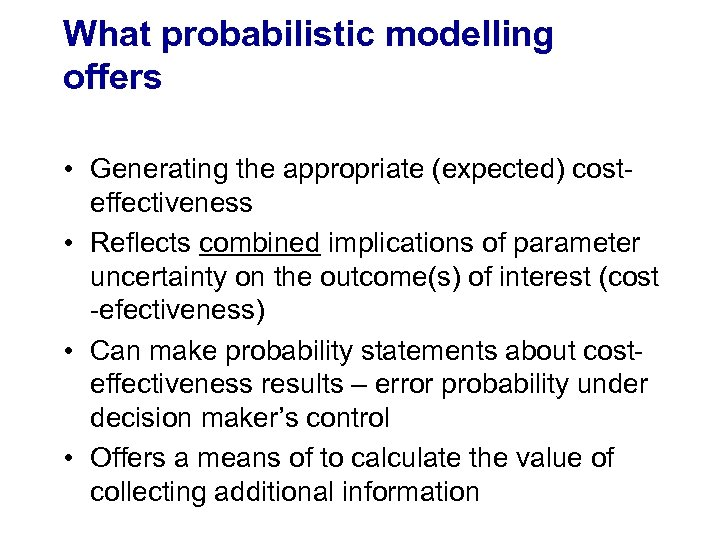What probabilistic modelling offers • Generating the appropriate (expected) costeffectiveness • Reflects combined implications of parameter uncertainty on the outcome(s) of interest (cost -efectiveness) • Can make probability statements about costeffectiveness results – error probability under decision maker’s control • Offers a means of to calculate the value of collecting additional information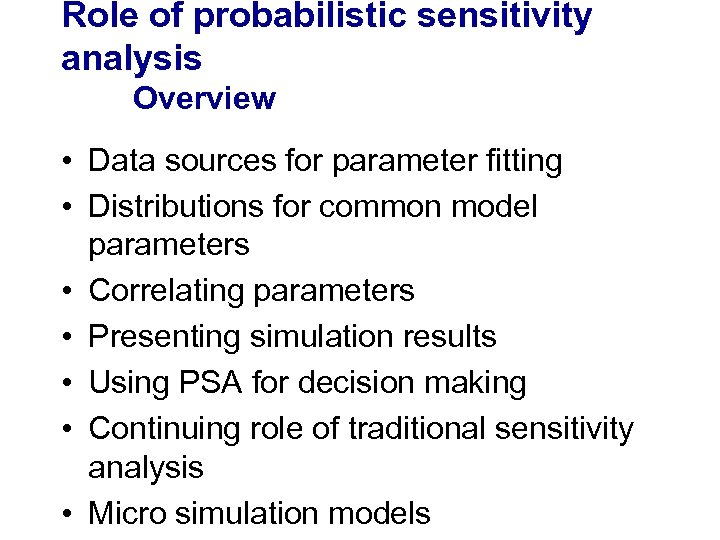Role of probabilistic sensitivity analysis Overview • Data sources for parameter fitting • Distributions for common model parameters • Correlating parameters • Presenting simulation results • Using PSA for decision making • Continuing role of traditional sensitivity analysis • Micro simulation models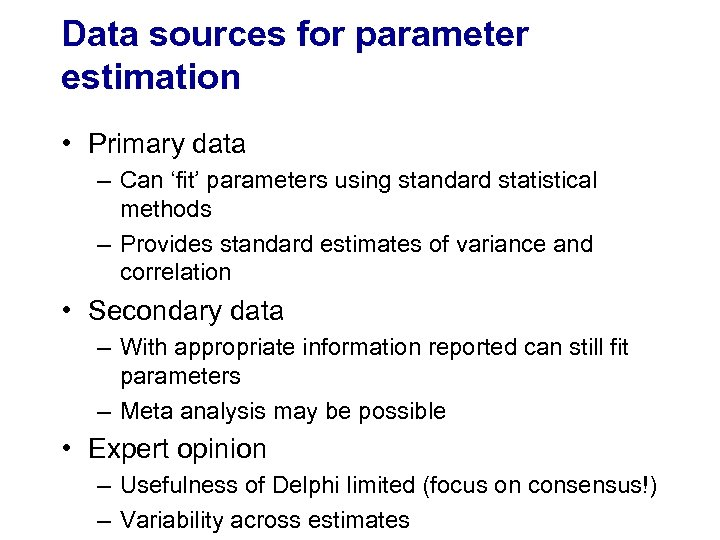Data sources for parameter estimation • Primary data – Can ‘fit’ parameters using standard statistical methods – Provides standard estimates of variance and correlation • Secondary data – With appropriate information reported can still fit parameters – Meta analysis may be possible • Expert opinion – Usefulness of Delphi limited (focus on consensus!) – Variability across estimates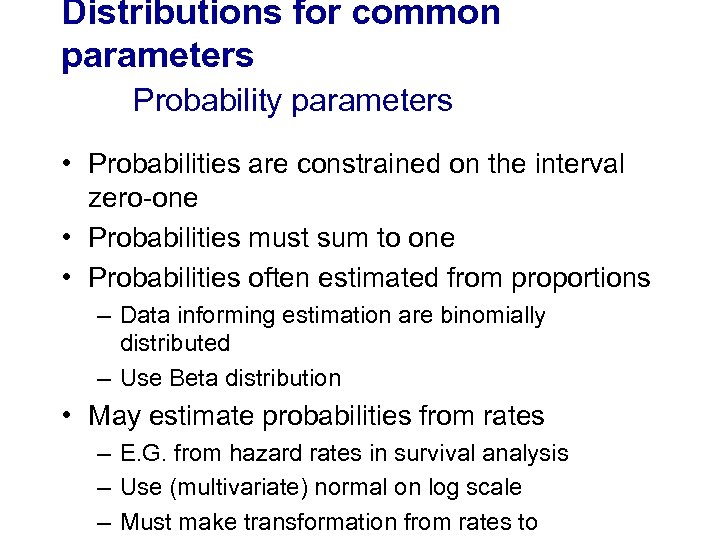Distributions for common parameters Probability parameters • Probabilities are constrained on the interval zero-one • Probabilities must sum to one • Probabilities often estimated from proportions – Data informing estimation are binomially distributed – Use Beta distribution • May estimate probabilities from rates – E. G. from hazard rates in survival analysis – Use (multivariate) normal on log scale – Must make transformation from rates to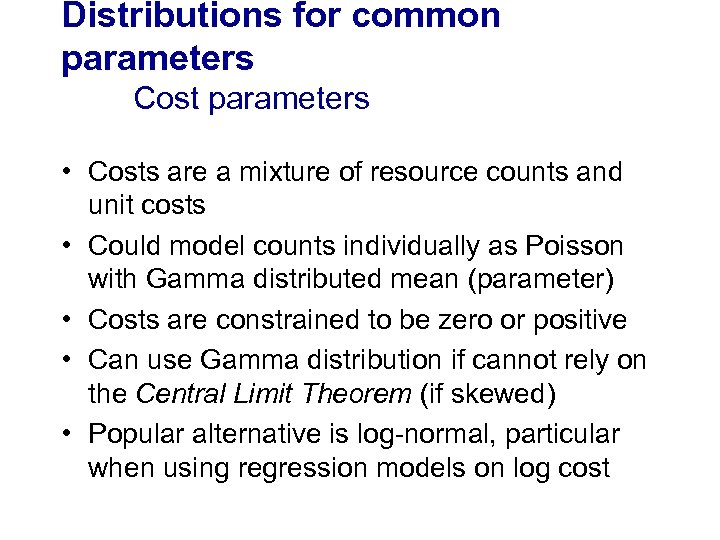Distributions for common parameters Cost parameters • Costs are a mixture of resource counts and unit costs • Could model counts individually as Poisson with Gamma distributed mean (parameter) • Costs are constrained to be zero or positive • Can use Gamma distribution if cannot rely on the Central Limit Theorem (if skewed) • Popular alternative is log-normal, particular when using regression models on log cost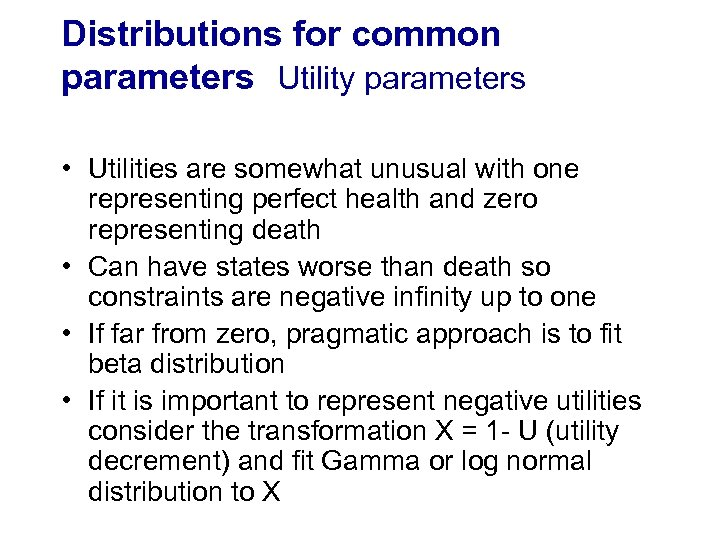Distributions for common parameters Utility parameters • Utilities are somewhat unusual with one representing perfect health and zero representing death • Can have states worse than death so constraints are negative infinity up to one • If far from zero, pragmatic approach is to fit beta distribution • If it is important to represent negative utilities consider the transformation X = 1 - U (utility decrement) and fit Gamma or log normal distribution to X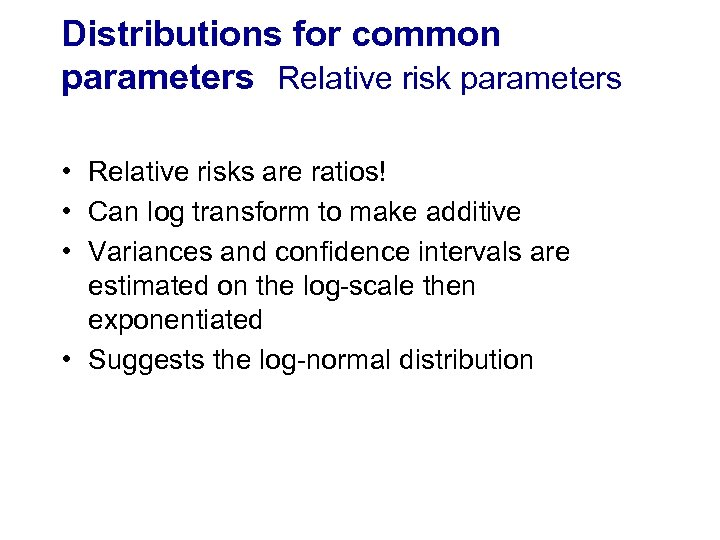Distributions for common parameters Relative risk parameters • Relative risks are ratios! • Can log transform to make additive • Variances and confidence intervals are estimated on the log-scale then exponentiated • Suggests the log-normal distribution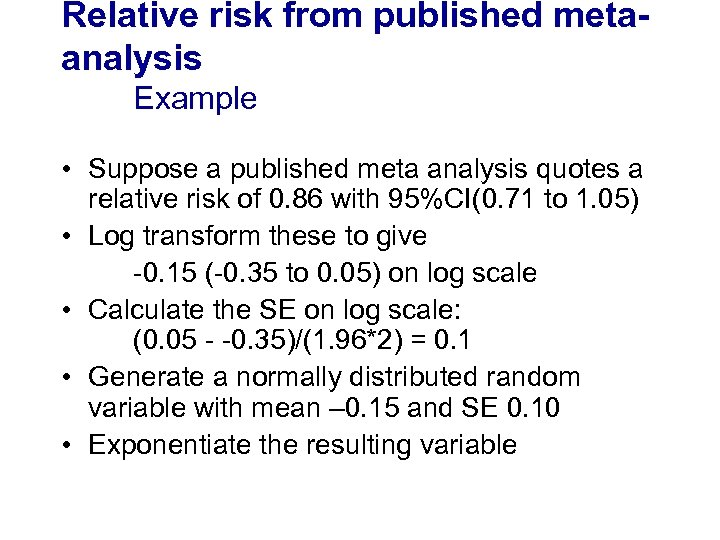Relative risk from published metaanalysis Example • Suppose a published meta analysis quotes a relative risk of 0. 86 with 95%CI(0. 71 to 1. 05) • Log transform these to give -0. 15 (-0. 35 to 0. 05) on log scale • Calculate the SE on log scale: (0. 05 - -0. 35)/(1. 96*2) = 0. 1 • Generate a normally distributed random variable with mean – 0. 15 and SE 0. 10 • Exponentiate the resulting variable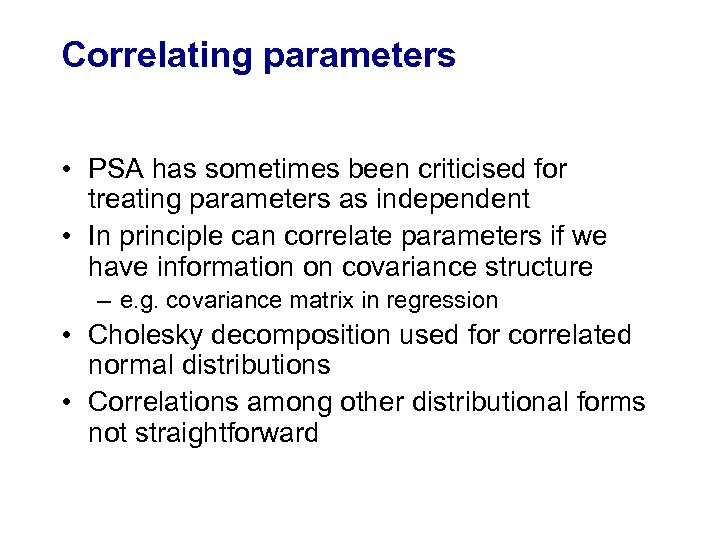Correlating parameters • PSA has sometimes been criticised for treating parameters as independent • In principle can correlate parameters if we have information on covariance structure – e. g. covariance matrix in regression • Cholesky decomposition used for correlated normal distributions • Correlations among other distributional forms not straightforward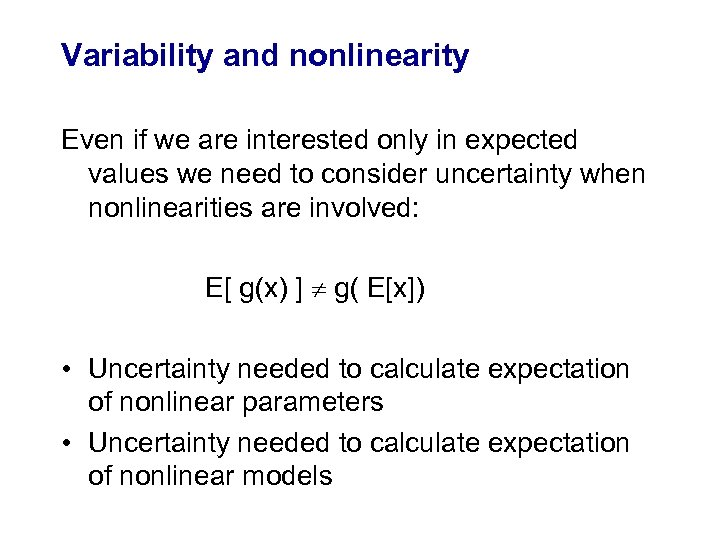Variability and nonlinearity Even if we are interested only in expected values we need to consider uncertainty when nonlinearities are involved: E[ g(x) ] g( E[x]) • Uncertainty needed to calculate expectation of nonlinear parameters • Uncertainty needed to calculate expectation of nonlinear models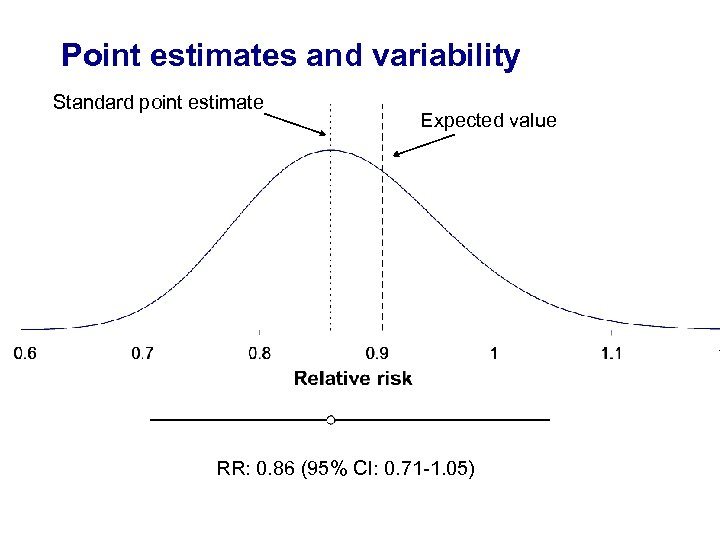Point estimates and variability Standard point estimate Expected value RR: 0. 86 (95% CI: 0. 71 -1. 05)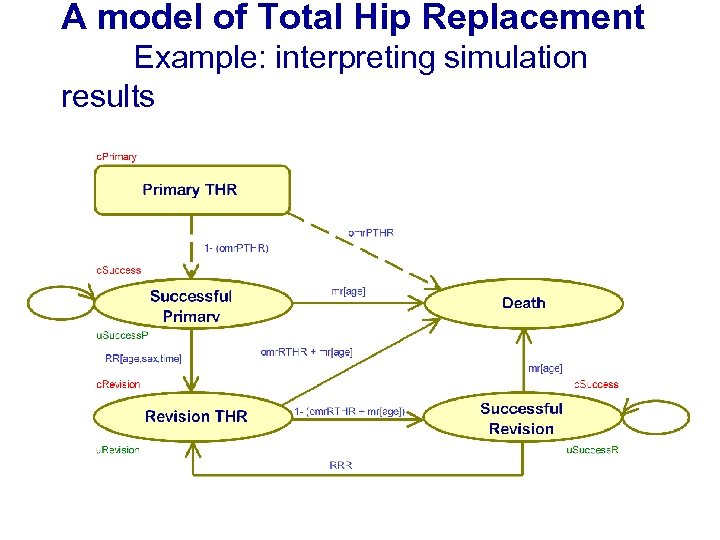A model of Total Hip Replacement Example: interpreting simulation results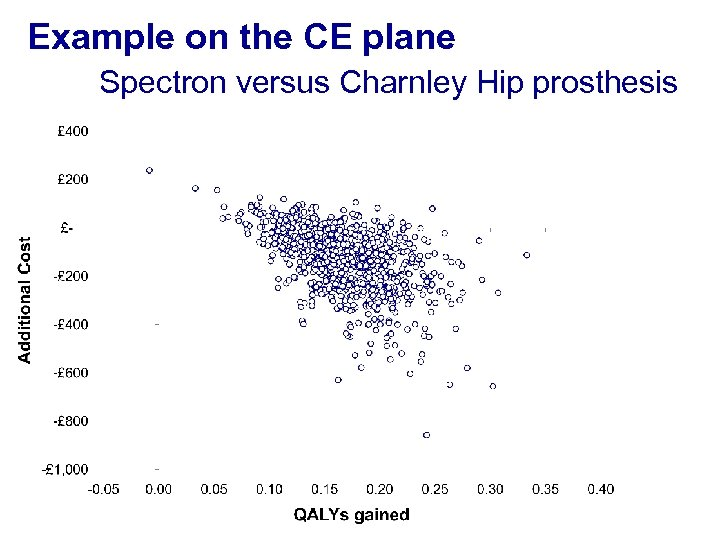Example on the CE plane Spectron versus Charnley Hip prosthesis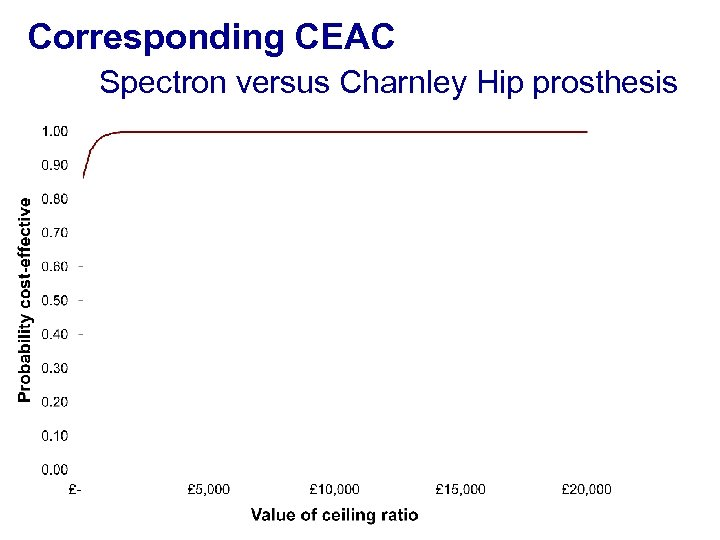Corresponding CEAC Spectron versus Charnley Hip prosthesis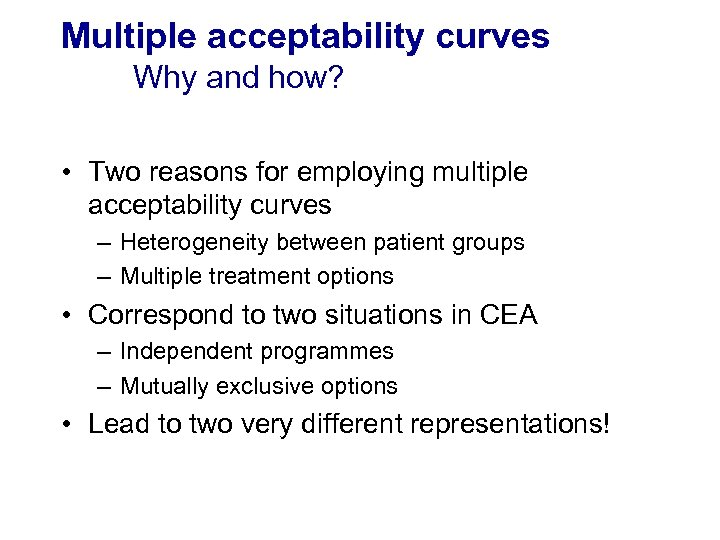Multiple acceptability curves Why and how? • Two reasons for employing multiple acceptability curves – Heterogeneity between patient groups – Multiple treatment options • Correspond to two situations in CEA – Independent programmes – Mutually exclusive options • Lead to two very different representations!Multiple CEACs: handling heterogeneity Spectron versus Charnley (Males)Multiple CEACs: handling heterogeneity Spectron versus Charnley (Females)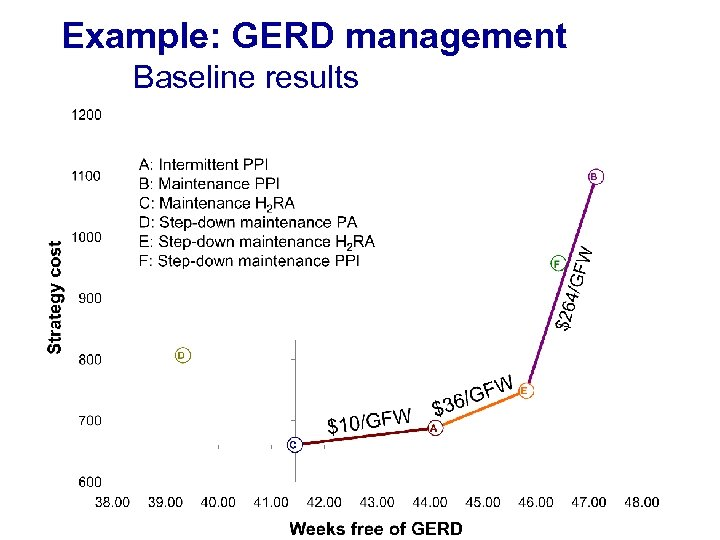Example: GERD management Baseline results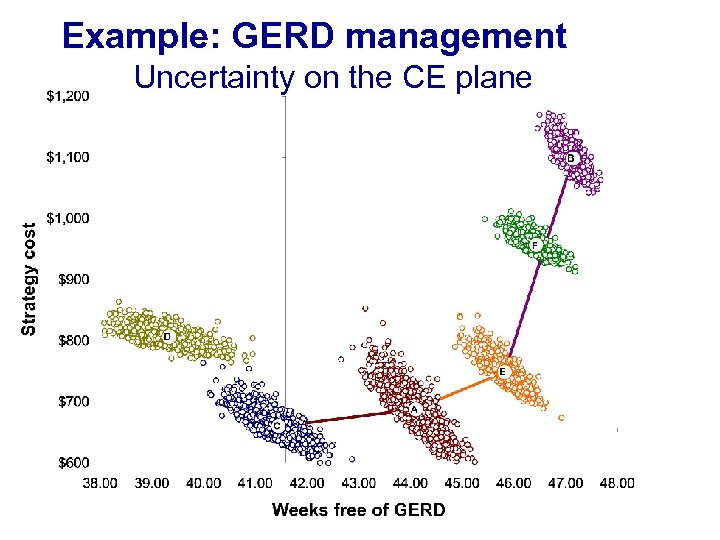Example: GERD management Uncertainty on the CE plane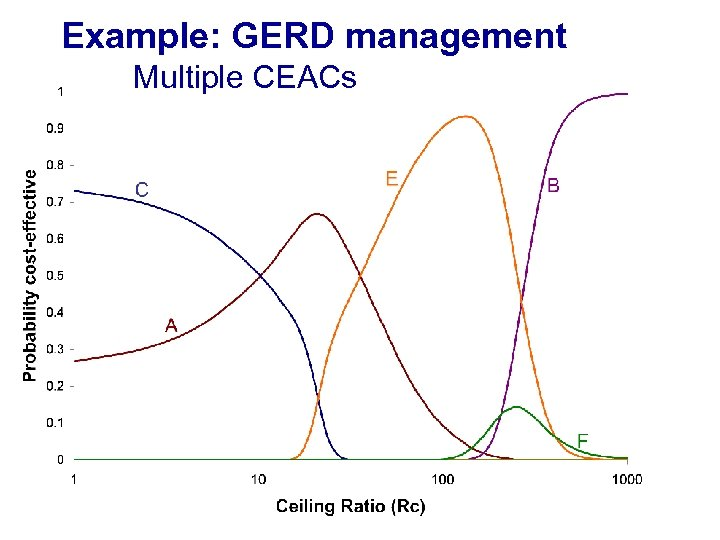Example: GERD management Multiple CEACs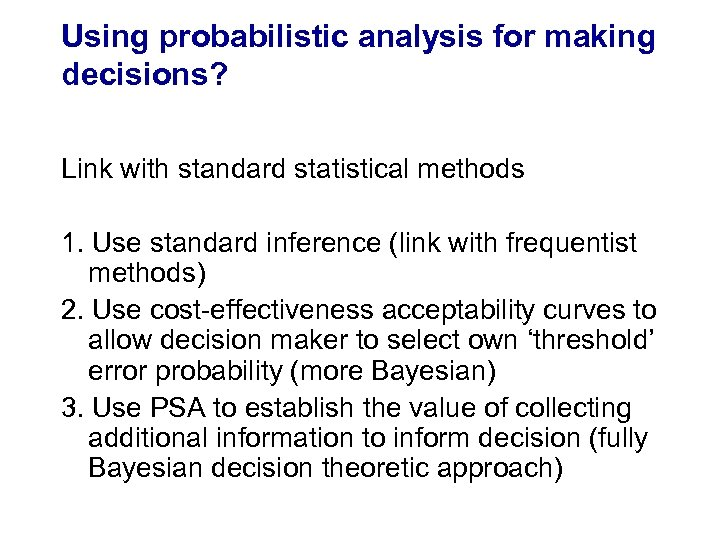Using probabilistic analysis for making decisions? Link with standard statistical methods 1. Use standard inference (link with frequentist methods) 2. Use cost-effectiveness acceptability curves to allow decision maker to select own ‘threshold’ error probability (more Bayesian) 3. Use PSA to establish the value of collecting additional information to inform decision (fully Bayesian decision theoretic approach)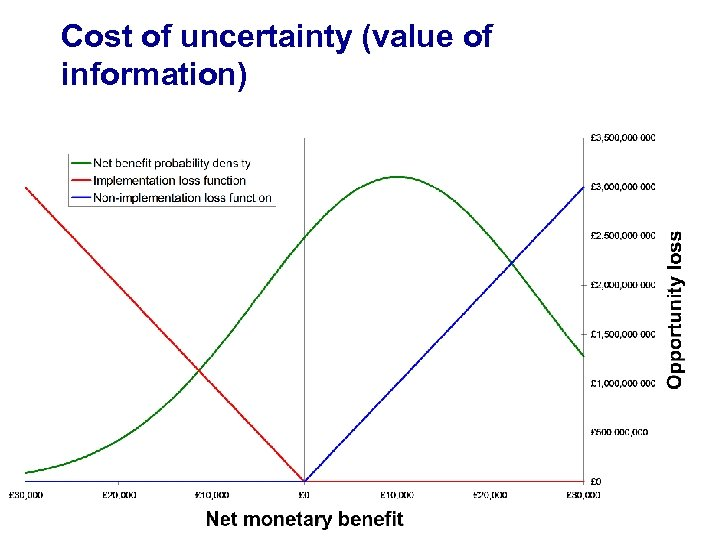Cost of uncertainty (value of information)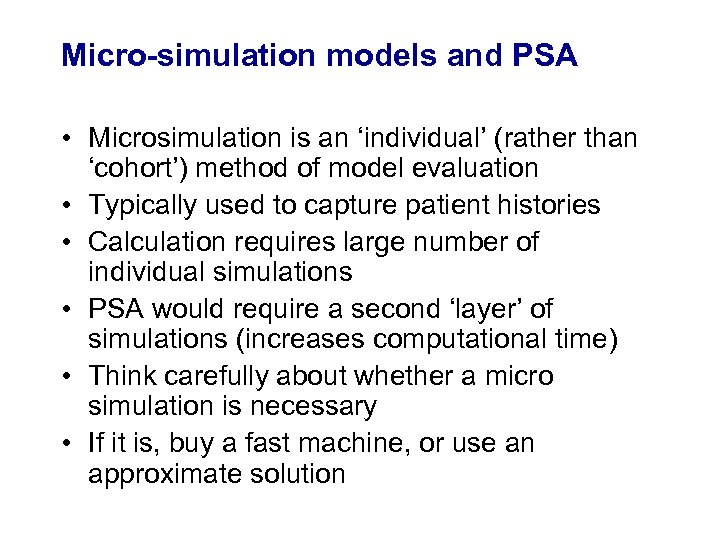Micro-simulation models and PSA • Microsimulation is an ‘individual’ (rather than ‘cohort’) method of model evaluation • Typically used to capture patient histories • Calculation requires large number of individual simulations • PSA would require a second ‘layer’ of simulations (increases computational time) • Think carefully about whether a micro simulation is necessary • If it is, buy a fast machine, or use an approximate solution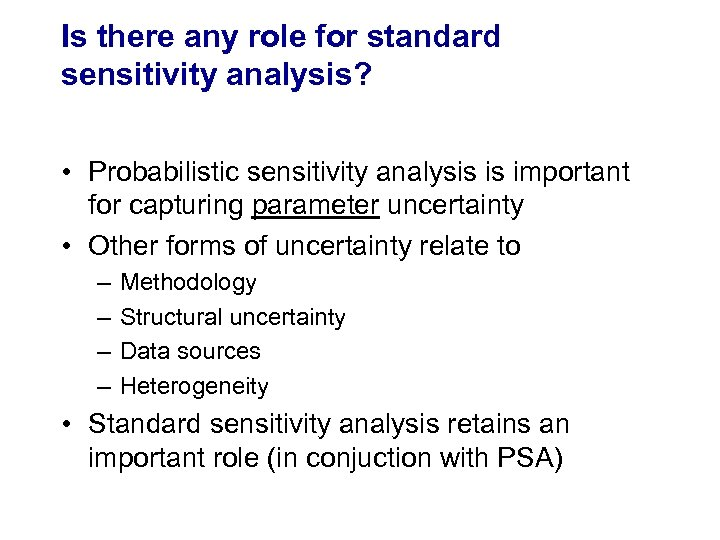Is there any role for standard sensitivity analysis? • Probabilistic sensitivity analysis is important for capturing parameter uncertainty • Other forms of uncertainty relate to – – Methodology Structural uncertainty Data sources Heterogeneity • Standard sensitivity analysis retains an important role (in conjuction with PSA)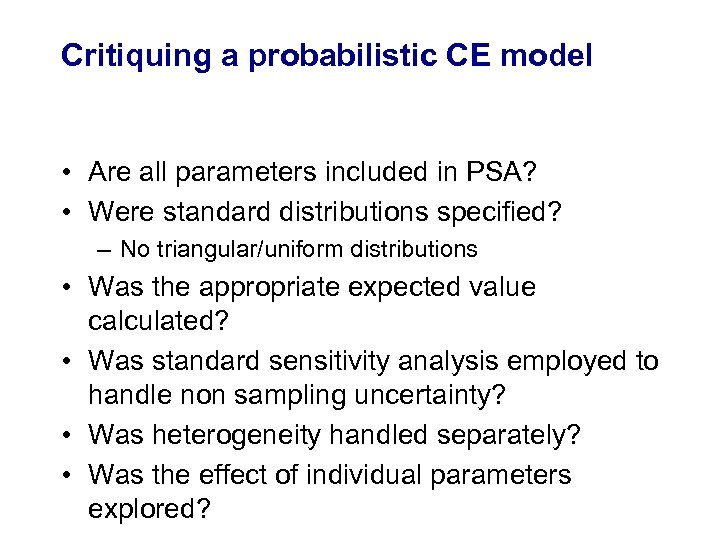Critiquing a probabilistic CE model • Are all parameters included in PSA? • Were standard distributions specified? – No triangular/uniform distributions • Was the appropriate expected value calculated? • Was standard sensitivity analysis employed to handle non sampling uncertainty? • Was heterogeneity handled separately? • Was the effect of individual parameters explored?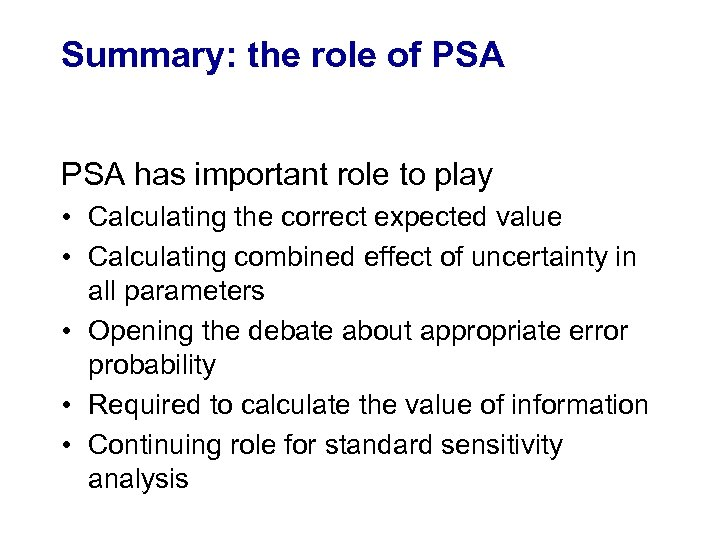Summary: the role of PSA has important role to play • Calculating the correct expected value • Calculating combined effect of uncertainty in all parameters • Opening the debate about appropriate error probability • Required to calculate the value of information • Continuing role for standard sensitivity analysis